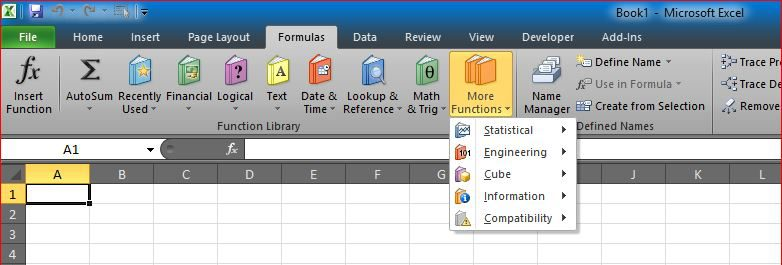# Text-to-Columns, dynamically using formulas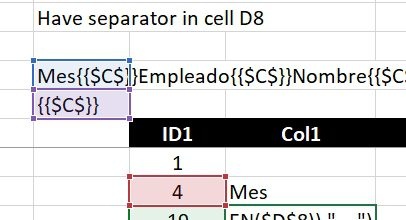I often use the technique of concatenating columns into 1 cell with separators.

Something like the CSV, 1 line that has all values for a single row (all columns for that row) into 1 text block.

And then, because of that, I need to extract that back, into table

I know there is “Text-to-Columns” in Excel, but I wanted that dynamically, so if source got changed, the columns output will be changed too.

So, here is the example, having three formulas in two columns.

Formula 1 in column E starting 11 down (Cell E10 just has the value of 1)

`=IFERROR(SEARCH(\$D\$8,\$D\$7,E10+1),"---")`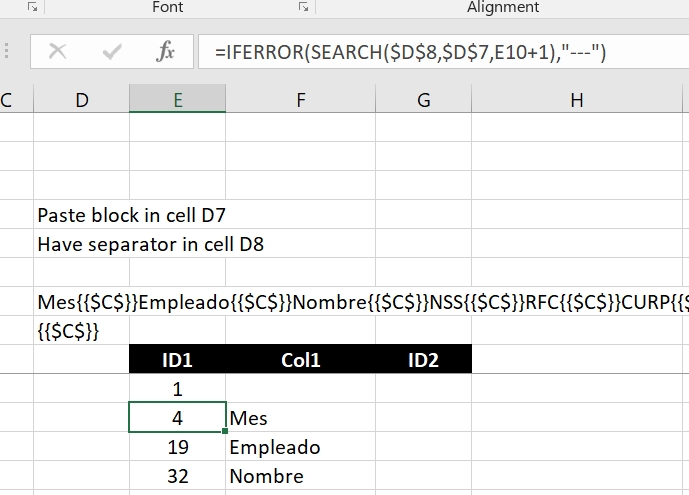Formula 2 in cell F11 only, to bring first item

`=IFERROR(MID(\$D\$7,E10,E11-1),"---")`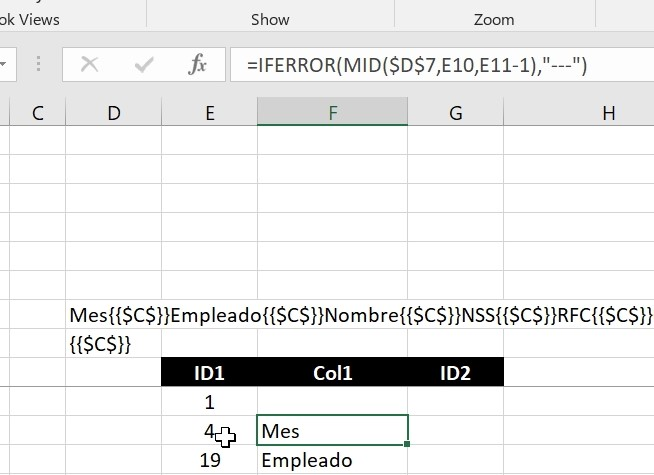Then, at last Formula 3, to get all additional columns from text block

`=IFERROR(MID(\$D\$7,E11+LEN(\$D\$8),E12-E11-LEN(\$D\$8)),"---")`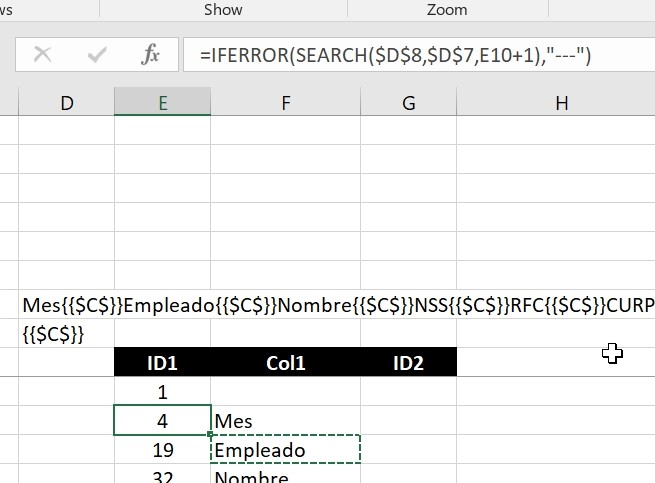I wanted to make it very clear and simple to show the case, data used in example is dummy data means nothing, just for sake of example.